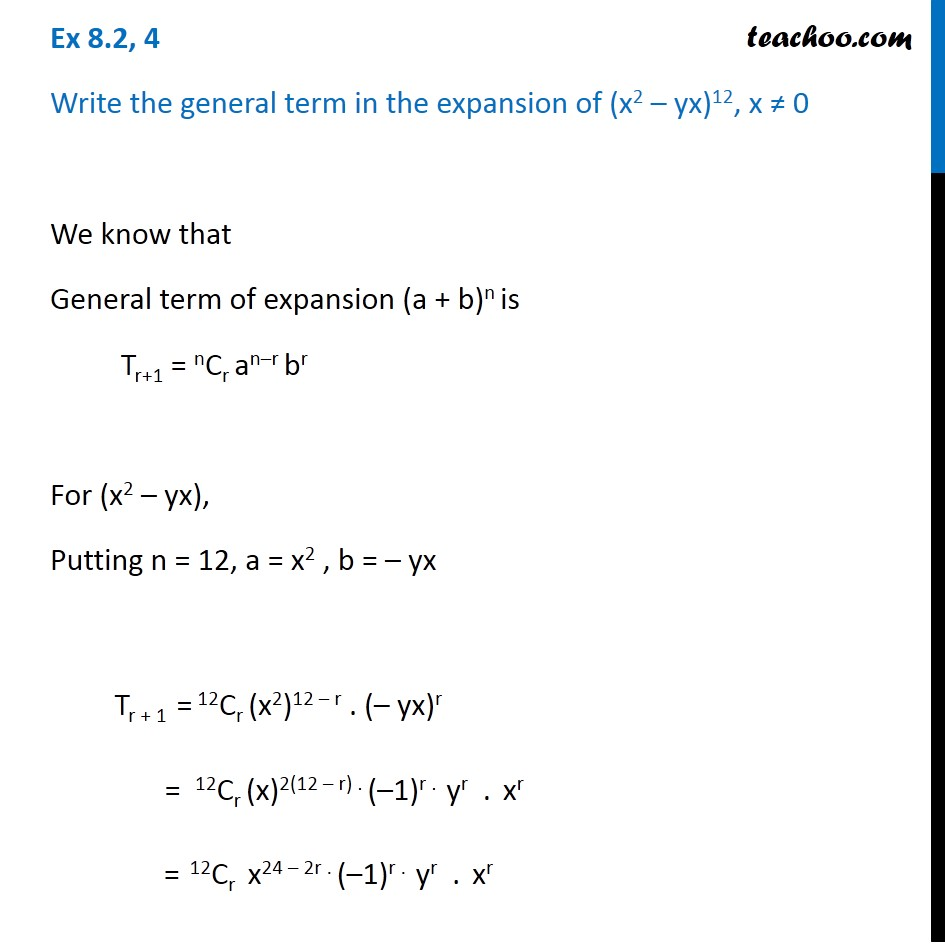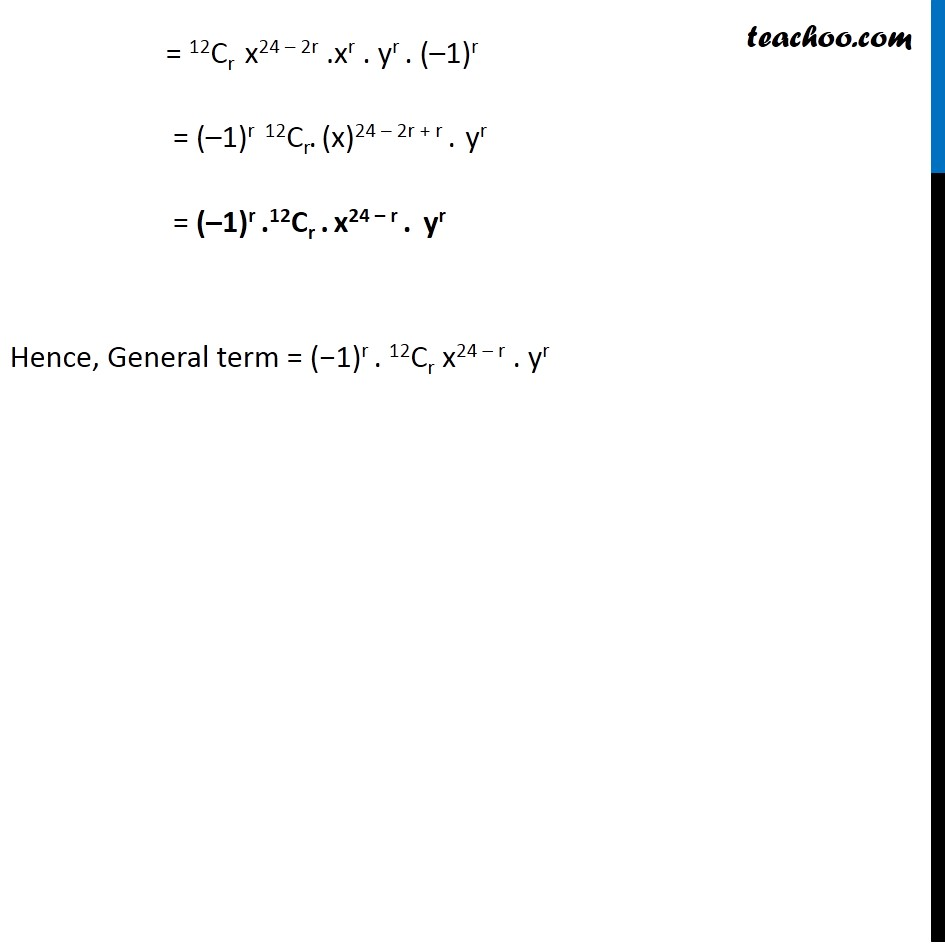General Term of Binomial Theorem

Chapter 7 Class 11 Binomial Theorem
Serial order wiseLearn in your speed, with individual attention - Teachoo Maths 1-on-1 Class

### Transcript

Question 4 Write the general term in the expansion of (x2 – yx)12, x ≠ 0 We know that General term of expansion (a + b)n is Tr+1 = nCr an–r br For (x2 – yx), Putting n = 12, a = x2 , b = – yx Tr + 1 = 12Cr (x2)12 – r . (– yx)r = 12Cr (x)2(12 – r) . (–1)r . yr . xr = 12Cr x24 – 2r . (–1)r . yr . xr = 12Cr x24 – 2r .xr . yr . (–1)r = (–1)r 12Cr. (x)24 – 2r + r . yr = (–1)r .12Cr . x24 – r . yr Hence, General term = (−1)r . 12Cr x24 – r . yr